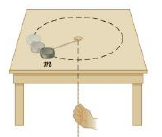Chapter 8, Problem 71P

Chapter
Section
Textbook Problem

The puck in Figure P8.71 has a mass of 0.120 kg. Its original distance from the center of rotation is 40.0 cm, and it moves with a speed of 80.0 cm/s. The string is pulled downward 15.0 cm through the hole in the frictionless table. Determine the work done on the puck. Hint: Consider the change in kinetic, energy of the puck.Figure P8.71

To determine
The work done on the puck.

Explanation

Given info: Mass of the puck is 0.120kg , initial radial distance of the puck is 40.0cm , initial translational speed of the puck is 80.0cm/s , and the final radius of the circular path of the puck is 25.0cm .

Explanation: The change in kinetic energy of the puck represents the work done on the puck that is Wnet=KEfKEi=(1/2)Ifωf2(1/2)Iiωi2 but the initial angular speed of the puck is defined as ωi=(vt)i/ri and final angular speed of the puck is determined by conservation of angular momentum of the puck such that ωf=(Ii/If)ωi=[(Ii/If)(vt)i/ri] and the initial and final moment of inertias of puck would be mri2 and mrf2 . By using all these expressions, the work done on the puck is calculated.

The formula for the work done on the puck is,

Wnet=m2[(rirf)21](vt)i2

• m is mass of the puck

Still sussing out bartleby?

Check out a sample textbook solution.

See a sample solution

The Solution to Your Study Problems

Bartleby provides explanations to thousands of textbook problems written by our experts, many with advanced degrees!

Get Started